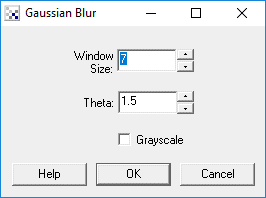# Gaussian Filter

Similar to the mean filter the Gaussian filter will smooth an image but will preserve edges better than the more basic mean filter.

The Gaussian filter gives more weight to the current pixel position and then tapers the weights as distance increases according to the Gaussian formula. By weighting a pixels contribution to the final pixel value this filter can better preserve edges than the mean filter which specifies equal weights to all pixels within the filter window.

The Gaussian Filter is a convolution filter whose convolution matrix is a Gaussian distribution. For a 1-D Gaussian filter the single filter values are defined as

G(x) = sqrt(1.0/(sqrt(2.0*PIE*theta)))*exp(-(x*x)/(2*theta*theta)))

For example the following charts represent a Gaussian filter of window size 7 and differing thetas.

 Theta = 1.00 5 24 39 24 5 0
 Theta = 1.53 10 21 26 21 10 3
 Theta = 2.06 12 17 19 17 12 6

Couple points to note:

1. The values have been scaled to add to 255.
2. Below theta 1.0 the filter can be reduced to size 5 since the endpoints have fallen off to zero.
3. As theta increases the filter becomes more flatter as neighboring pixels are given more weight.

Thus, if theta is less than 0.5, the filter becomes a single point (and not very useful). Likewise, if theta is greater than the (window size)/6, then the filter becomes more like a mean filter.

This filter is applied to an image in a two phase approach. First the horizontal direction is filtered using the above filter in a similar manner to a convolution filter by taking each pixel in the image, centering the filter on that pixel (the middle value) and then multiplying the pixel values by the weight at each filter location followed by a final divide to get the resulting new pixel value. This process is then repeated vertically on the horizontally processed image to create the final image.

The Gaussian filter is one of the more popular blurring filters as it has its basis in the human visual perception system. It has been found that neurons create a similar filter when processing visual images.

## Interface## Instructions

1. Window Size - Specify the window size of the filter. This is often referred to as the kernal size. Larger windows require more processing but can achieve higher levels of blurring.

2. Theta - Specify the theta or sigma of the filter. This controls the relative weighs or effect the surrounding pixels have on the final pixels. Smaller theta results in more weight on the center pixel and results in less blurring, larger theta causes less weight on the center pixel and causes more blurring.

3. Grayscale - To increase speed, you can select the grayscale option which will only examine the green channel to produce the blur results.

## Example

 Source Gaussian Blur